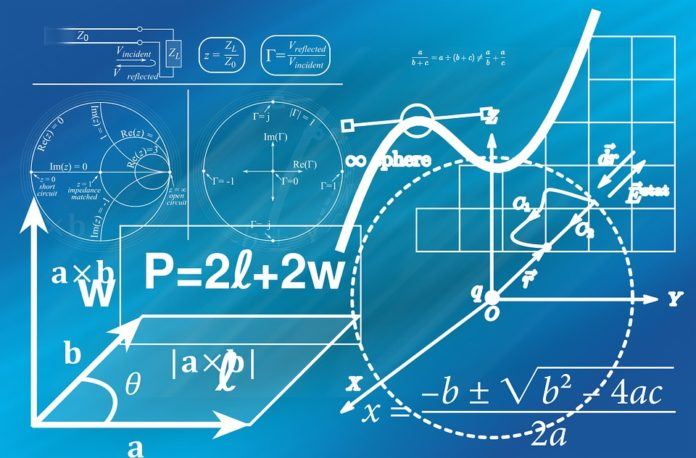# Maximum Likelihood Estimation

0
1183Maximum Likelihood Estimation often called as MLE, is used for estimating the parameters of a statistical model when certain observations are given. Before we start learning more about this topic, let me present you the prerequisites for studying Maximum Likelihood Estimation. They are:

• Probability and Random Processes
• Basics of Calculus

### Introduction

Suppose we have some data points drawn from a normal distribution. But the question is: which normal distribution? Because a normal distribution is not a single distribution, we have a different distribution for each pair of and. A particular binomial distribution is represented by the two parameters and, and a particular exponential distribution is obtained by. These types of distributions are called parametric distributions.

For example, a linear model is described as where are the parameters for this specific parametric model.

Most of the times, we know that particular random data are obtained from particularly known distributions, whose parameters are unknown. For example, the time required by students to answer a particular question follows a Bernoulli distribution with unknown parameter. In this case, we can use the data to find the possible value of the parameter, and by using this method we can predict the time required to answer. Similarly, lottery systems follow a normal distribution to decide the winner, and we can use previous data of lottery systems as observations to draw inferences about the values of the parameters and. So by learning the distribution, you can even increase your chances of winning the lottery.

### Maximum Likelihood EstimationWe generally find the probability of data from a model with known parameters. But now, we have to approximate the probability of parameters of a given parametric model and its observed data. So, indirectly we have to find the answer to this question: For which parameter value the observed data have the biggest probability? Maximum likelihood estimation (MLE) helps us answer this question.

Definition: The data which is assumed to be the maximum likelihood estimate (MLE) for the parameter is the value of that maximizes the likelihood. That is, the MLE is the value of for which the data have the biggest probability.

Assume we have for which probability density function of can be written as, then, the joint pdf of which we’ll call is the product of the individual pdfs (assuming events are independent to each other):

Now, as we already got the basic idea of maximum likelihood estimation, we can treat the “likelihood function“ as a function of, and find the value of θ that maximizes it.

Is this getting boring? Too much mathematical formulas? Let’s take a simple example to understand the whole idea of MLE and how it is applied to actual data.

#### Example:

Let’s assume that the total scores of randomly selected IIT Bombay students follow an exponential distribution with a parameter, which is unknown. A random sample of 5 IIT Bombay students yielded the following total score (out of 200):

115 122 130 127 149
What is the MLE for?

Solution:Let be the total score obtained by the student and let be the value taken by. Then each has a pdf.
We assume the total scores of the students are independent, so the joint pdf is the product of the individual pdfs of each student:

where , , , ,

=

It is often easier to work with the natural log of the likelihood function. Since the maxima of the likelihood and log likelihood coincide, we will get the same answer in case of both.

Finally, we use the first derivative to find maxima of the function in turns MLE:

And here we have our maximum likelihood estimate for.

1. In this example, we have used a capital letter for a random variable which is an estimator and the corresponding lowercase letter for the value it taken by that random variable, that is, the value of this variable is fixed and taken based on an obtained sample. This will be the usual practice.
2. The MLE is a statistic operation as it is obtained from the data.
3. You should always confirm that the obtained point is maxima by using the second derivative test.

It is not necessary to follow this exact method to find maxima of likelihood. Here we use the first derivative to find maxima, but in most of the cases, in a real-world problem, the derivative of the log-likelihood function is very hard to analytically deal with, that is, manually finding derivative for this function is too hard or nearly impossible. Therefore, iterative methods are used to find solutions for the parameter estimates. For example, the expectation maximization algorithm. However, the overall problem remains the same, that is, to find a critical point of maxima for the given likelihood function.

Maximum Likelihood Estimation (MLE) by using log-likelihood is a very basic algorithm for parameter estimation for any model or distribution. Let’s have a look at a couple of drawbacks of Maximum Likelihood Estimation as it is also important to know the downside of a particular algorithm. For a smaller amount of data, MLE does not give a precise (right) solution. If we have small numbers of observations (say 15 to 20), the solution given by MLE will be heavily biased. Sometimes it takes high computation power mainly while finding the critical point for maxima. With infinite data, MLE can estimate the optimal parameter for the model, and approximate it well for small but robust datasets.Machine Learning Basics: Building Regression Model in Python’ is an excellent course if you wish to try out your career at Machine Learning as well. With topics such as Data Exploration, Missing Value Imputation, and correlation analysis, you’ll be able to have your fundamentals cleared.

In this article, we learned about Maximum Likelihood Estimation. Hope you found it useful. Stay tuned for more amazing articles!